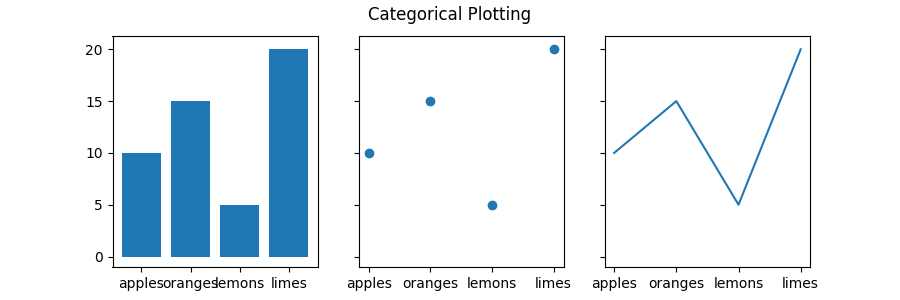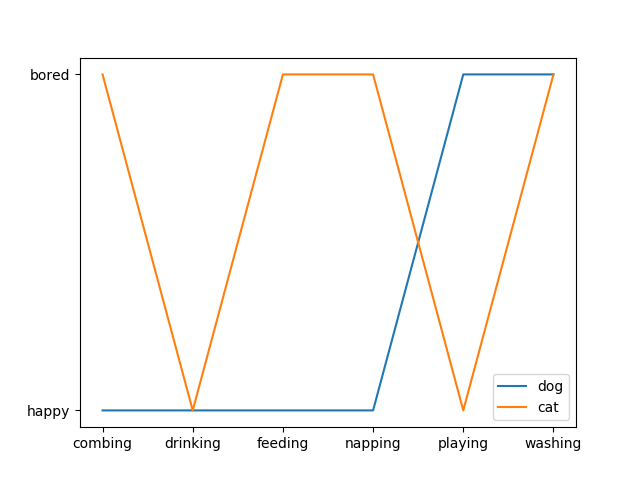# Plotting categorical variables¶

How to use categorical variables in Matplotlib.

Many times you want to create a plot that uses categorical variables in Matplotlib. Matplotlib allows you to pass categorical variables directly to many plotting functions, which we demonstrate below.

import matplotlib.pyplot as plt

data = {'apples': 10, 'oranges': 15, 'lemons': 5, 'limes': 20}
names = list(data.keys())
values = list(data.values())

fig, axs = plt.subplots(1, 3, figsize=(9, 3), sharey=True)
axs.bar(names, values)
axs.scatter(names, values)
axs.plot(names, values)
fig.suptitle('Categorical Plotting')Out:

Text(0.5, 0.98, 'Categorical Plotting')


This works on both axes:

cat = ["bored", "happy", "bored", "bored", "happy", "bored"]
dog = ["happy", "happy", "happy", "happy", "bored", "bored"]
activity = ["combing", "drinking", "feeding", "napping", "playing", "washing"]

fig, ax = plt.subplots()
ax.plot(activity, dog, label="dog")
ax.plot(activity, cat, label="cat")
ax.legend()

plt.show()Keywords: matplotlib code example, codex, python plot, pyplot Gallery generated by Sphinx-Gallery